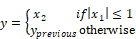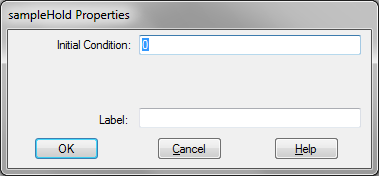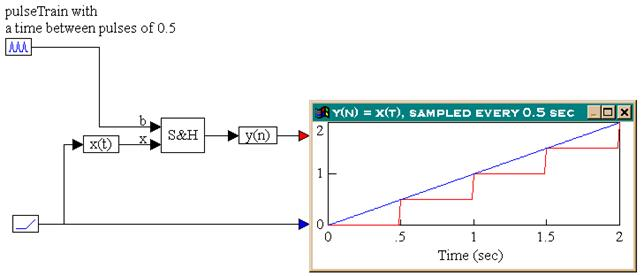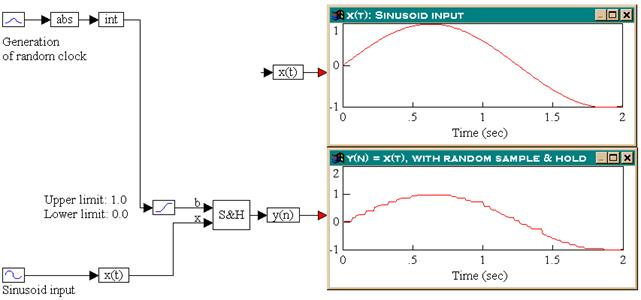### sampleHoldThe sampleHold block latches an input value under the control of a clock signal, x1, which is represented as Boolean input b. When b is true, input signal x2, which is represented as input x, is sampled and held until b is true again. Boolean inputs can be regularly or irregularly spaced.Initial Condition: Indicates the initial condition for the sampleHold. The default is zero.

Label: Indicates a user-defined block label.

#### Examples

1. Sample and hold with regularly-spaced clock

Consider the equation:

y(n) = x(t)

sampled every 0.5s. Furthermore, let x(t) be a ramp signal. This system can be realized as shown below.As seen in the plot block, the first clock pulse occurs at 0.5s. Until this time, the output of the sampleHold block is zero. At 0.5s, the input signal is sampled and the value is used as output for the sampleHold block. The output of the sampleHold block is held at this value until the occurrence of the next clock pulse at 1.0s. At this time, the input signal is again sampled and the new value is presented to the output of the sampleHold block, and the process repeats itself.

2. Sample and hold with irregularly-spaced clock

Consider the equation:

y(n) = x(t)

sampled randomly. Furthermore, let x(t) be a sinusoid signal with a frequency of 2.5 rad/s. This system can be realized as shown below.A sinusoid block with a frequency of 2.5 rad/s generates the sinusoid signal and a gaussian block produces a randomly varying signal. The randomly varying signal is converted to a random clock by taking the absolute value of the random signal and then using only the integer portion of it. The output of the int block is passed through a limit block to restrict the signal to the range (0, 1). The output of the limit block is connected to the top input of the sampleHold block. The output of the sampleHold block is connected to the variable y(n), which is connected to a plot block. The actual input, x(t) is monitored separately in another plot block.

By comparing the outputs in the two plot blocks, the output of the sampleHold block is a randomly sampled and held version of the input sinusoid.